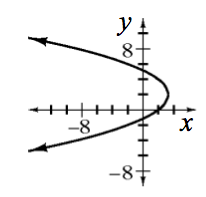### Home > A2C > Chapter Ch11 > Lesson 11.3.1 > Problem11-128

11-128.
1. For each equation below, identify the shape of the graph, list all of the necessary information, and sketch a graph. Homework Help ✎

1.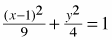2. (x − 4)2 + (y + 2)2 = 25

3.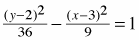4. 3x + (y − 2)2 = 10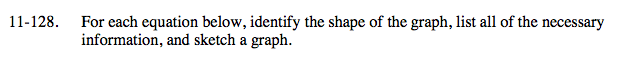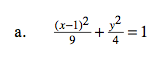What does the parent graph look like? Notice the terms are added.

What is the center? Is the major axis vertical or horizontal?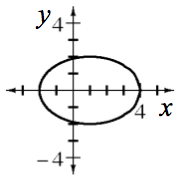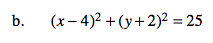This equation has the form (xh)2 + (yk)2 = r2. What is this shape?

What is the center and radius?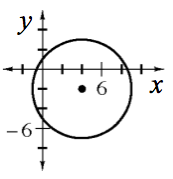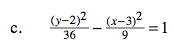What does the parent graph look like? Notice the terms are subtracted.

Find the center, foci, and vertices.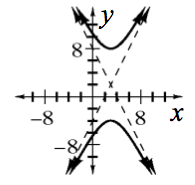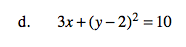When you isolate the x term, the equation becomes:

$x=-\frac{1}{3}(y-2)^2+\frac{10}{3}$

This is a sleeping parabola.